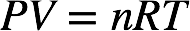# Problem: Acrylic acid (CH2=CHCOOH) is used to prepare polymers, adhesives, and paints. The first step in making acrylic acid involves the vapor-phase oxidation of propylene (CH2=CHCH3) to acrolein (CH2=CHCHO). This step is carried out at 330°C and 2.5 atm in a large bundle of tubes around which a heat-transfer agent circulates. The reactants spend an average of 1.8 s in the tubes, which have a void space of 100 ft3. How many pounds of propylene must be added per hour in a mixture whose mole fractions are 0.07 propylene, 0.35 steam, and 0.58 air?

###### FREE Expert Solution

The ideal gas law is given by:where P = pressure in atm, V = volume in L, n = moles of gas, R = universal gas constant, and T = temperature in Kelvin.

We can use the ideal gas law to calculate the total moles of gas present. We're given:
P = 2.5 atm          T = 330˚C + 273.15 = 603.15 K          V = 100 ft3

94% (326 ratings)###### Problem Details

Acrylic acid (CH2=CHCOOH) is used to prepare polymers, adhesives, and paints. The first step in making acrylic acid involves the vapor-phase oxidation of propylene (CH2=CHCH3) to acrolein (CH2=CHCHO). This step is carried out at 330°C and 2.5 atm in a large bundle of tubes around which a heat-transfer agent circulates. The reactants spend an average of 1.8 s in the tubes, which have a void space of 100 ft3. How many pounds of propylene must be added per hour in a mixture whose mole fractions are 0.07 propylene, 0.35 steam, and 0.58 air?